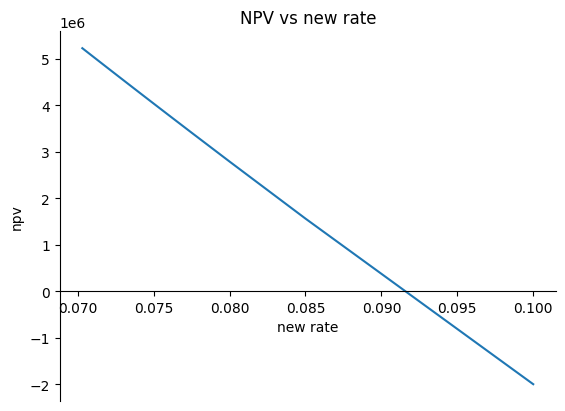# Refinancing notebook, Julia version¶

The following is a Julia version of the core parts of our refinancing calculations, for those of you interested in comparing Python with Julia.

In :
# Parameters

rnow = 0.10 # current interest rate, in yearly terms
term= 60 # remaining time to maturity in months
bnow= 100_000_000 # outstanding principal
rnew = 0.09 # new rate
penalty = 0.02 # prepayment penalty

using NPFinancial # same as Python's Numpy_Financial
using Printf

# define an npv function

function npv_refi(rnew, term, bnow, rnow, penalty)
oldpayment=pmt(rnow/12,term,-bnow)
newpayment=pmt(rnew/12,term,-bnow)
return pv(rnew/12,term,-(oldpayment-newpayment))-penalty*bnow
end

@printf("The NPV of refi is %.2f. ", npv_refi(rnew, term, bnow, rnow, penalty))

The NPV of refi is 354182.11.
In :
# Now we look for the breakeven refi rate
# graphically first
# then we use Julia's optimization tools

# a range of candidate interest rates

rnewrange=[(7+3/(100-i))/100 for i in 1:100]
npvrange=[npv_refi(r, term, bnow, rnow, penalty) for r in rnewrange]

#ax=gca()
#ax[:spines]["left"][:set_position]("zero")
using PyPlot

plot(rnewrange,npvrange)
ax1 =gca()
ax1.spines["bottom"].set_position(("data",0)) # moves the bottom axis to the center
ax1.spines["right"].set_color("none")
ax1.spines["top"].set_color("none")
ax1.xaxis.set_ticks_position("bottom") # Set the x-ticks to only the bottom
ax1.set_title("NPV vs new rate")
ax1.set_xlabel("new rate")
ax1.set_ylabel("npv")Out:
PyObject Text(24.000000000000007, 0.5, 'npv')
In :
using Roots

f(r)=npv_refi(r, term, bnow, rnow, penalty)   # I love this easy syntax in julia, like it even better than Python's and Matlab's lambda version

@printf("The breakeven reate is %.2f%%. ", 100*find_zero(f,0.1)) # how easy is this? Amazeballs

The breakeven reate is 9.15%.